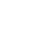# Calculate a sum using reduce in ImmutableJS

The reduce function in ImmutableJS allows you to transform and create new data from an existing data structure. In this example, we will do some math and calculate a sum based on data in an ImmutableJS Map.Taylor Bellinstructor
~ 5 years ago

In this example, I'm returning an assignment with `+=` :

`const subtotal = cart.get('items').reduce((t, i) => t += i.get('price'), 0)`

This code works, but it's not necessary to do the assignment, since the sum is what I actually want: `const subtotal = cart.get('items').reduce((t, i) => t + i.get('price'), 0)`